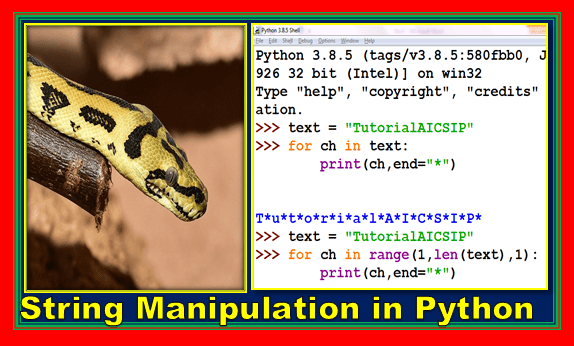String manipulation in Python is an important topic for python programming or computer science class 11 and Informatics Practices class 11.

Topics Covered

## Introduction to String Manipulation in Python

As you are aware that python allows to use following built-in data types:

1. Numbers
2. Strings
3. List
4. Tuple
5. Dictionary

So you have basic ideas about strings. A string data type is representing text values in the program. If you are accepting anything in single or double or triple quotes in python is known as a string.

It can be set of letters, symbols or numbers also.

Moreover you are also familiar with input and output operations as well. So let’s explore the string manipulation in python with some more operators and functions.

## Accessing a string through loop

As we have discussed a string is a collection of letters, numbers, and symbols each entity has its own position in the string. This is called an index. So using by using these indices you can access the string trough a loop.

Accessing a string by its index letter by letter is also known as traversing.

### Accessing string through for loop

To access the string letter by letter for loop can be used. Take a look at the following code:

``````>>> text = "TutorialAICSIP"
>>> for ch in text:
print(ch,end="*")

The output will be:
T*u*t*o*r*i*a*l*A*I*C*S*I*P*``````

### Accessing string through the loop using the range function

``````text = "TutorialAICSIP"
>>> for ch in range(0,len(text),1):
print(text[ch],end="*")

The output will be:
T*u*t*o*r*i*a*l*A*I*C*S*I*P*``````

When you want to access the string using range function another built-in function len() will be used to identify the length of a string.

### Reverse a string

``````>>> text ="TutorialAICSIP"
>>> l =len(text)
>>> for ch in range(-1,(-l-1),-1):
print(text[ch],end="")

The output will be:
PISCIAlairotuT``````

## String Manipulation in Python using Basic Operators

As you have used basic arithmetic operators + and * for “addition” and “multiplication” respectively.

These operators can be used for string manipulation in python as well. Here in string manipulation these operators are known as “concatenation” and “replication” operator.

### String concatenation operator “+”

It is used to join two words. Observe the below given code and output:

``````>>> text ="Tutorial" + "AI-CS-IP"
>>> print(text)
The output will be:
TutorialAI-CS-IP
``````

Valid combinations for + operator:

1. n + n = 3 + 3 = 6
2. s + s = “3” + “3” = 33

Invalid combinations:

1. n + s = 3 + “3”
2. s + n = “3” + 3

### String replication operator “*”

It is used to replicate the string number of time as specified in the expression. You can use number * string or string * number as well.

``````>>> text ="TutorialAICSIP"
>>> print(2*text,"-->",text*2)
The output will be:
TutorialAICSIPTutorialAICSIP --> TutorialAICSIPTutorialAICSIP``````

Valid combination for * operator:

1. n * n = 3 * 3 = 9
2. s * n = “\$” * 3 = “\$\$\$”
3. n * s = 3 * “\$” = “\$\$\$”

Invalid combinations:

1. s * s = “3” * “3”

Some facts:

1. Numbers combination returns the multiplication.
2. String combination will error in “*” operator.

Note: This operator can operate either string or number only, not both together.

## String Manipulation in Python using Membership Operators

There are two membership operators supported for string manipulation in python.

1. in
2. not in

### The “in” operator

As you have already used “in” operator with for loop. It us used to check the specified values is available there in specified string or not. It returns True if the text is found otherwise returns False.

``````text ="TutorialAICSIP"
if "Tutor" in text:
print("Tutor found")
else:
The output will be:
Tutor found``````

### The not in Operator

It is used reversed than “in” operator. Returns True when specified text is not available in the text, other wise False.

``````text ="TutorialAICSIP"
if "Tutor" not in text:
else:
print("Tutor found")
The output will be:

### String Manipulation in Python using comparison Operators

#### Using ASCII values Python code

Python provides ord() function to know the ASCII value of the specified character.

Ex.: ord(‘D’) returns 68

If you want to know the character from ASCII code then python provides chr() function.

Ex. chr(98)returns ‘b’

## Slices in String

Slices is another advanced feature supported by python. It will return the specific part from the given string and ranges. It will take the form of str[n1:n2].

``````>>> text="TutorialAICSIP"
>>> print(text[0:5])
Tutor
>>> print(text[3:9])
orialA
>>> print(text[:5])
Tutor
>>> print(text[5:])
ialAICSIP
>>> print(text[-1:])
P
>>> print(text[:-1])
TutorialAICSI
>>> print(text[-5:-4])
I
>>> print(text[1:3:3])
u``````